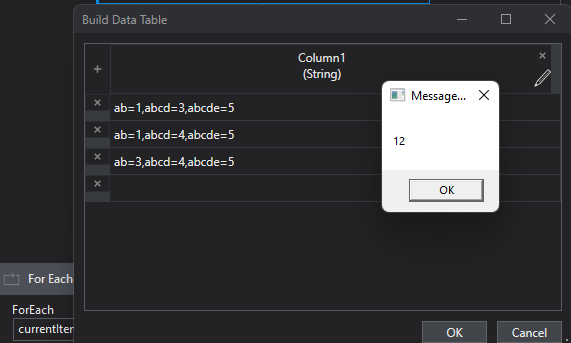# Datatablesum

Hi…all

I need to sum the column from the datatable needs to check which row is higher
Ex : row 1 ab=1,abcd=3,abcde=5
Row 2 ab=1,abcd=4,abcde=5
Row 3 ab=3,abcd=4,abcde=5

In this row 3 higher like that

can your requrement reformulated as following:

Input: datatable with three columns, sample data as above
Output: datarow, where the sum of all columns is max

HiThis is one line solution working for me
row with largest sum:

``````dataTable.AsEnumerable.OrderBy(function(x) Cint(x("Column1"))+Cint(x("Column2"))+Cint(x("Column3"))).Last
``````

Value of this sum:

``````dataTable.AsEnumerable.Select(function(x) Cint(x("Column1"))+Cint(x("Column2"))+Cint(x("Column3"))).Max
``````

Checkout this sample workflow
Main.xaml (14.9 KB)Regards
Sudharsan